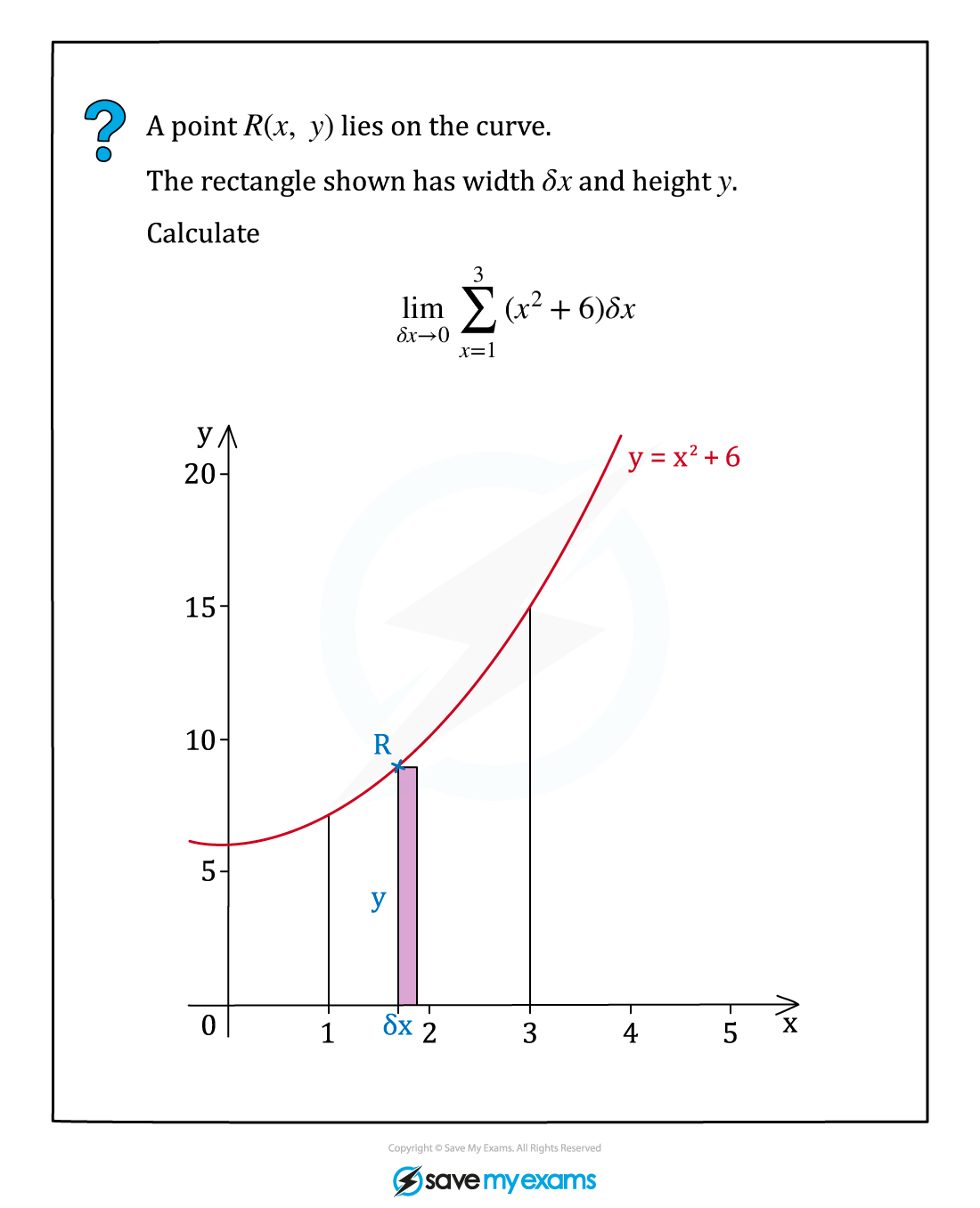# AQA A Level Maths: Pure复习笔记8.2.1 Integration as the limit of a sum

### Integration as the limit of a sum

#### Finding the area under a curve

• Definite integration allows us to find the area under a curve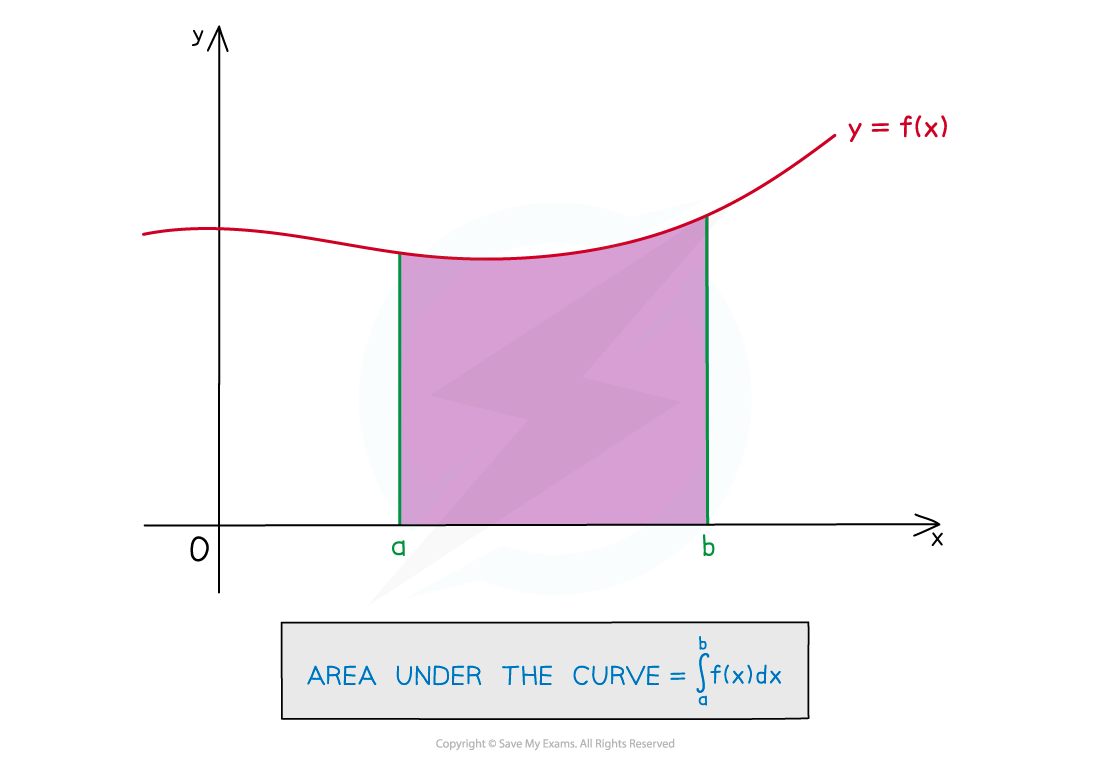•  An estimate for the area under the curve is the sum of the rectangular areas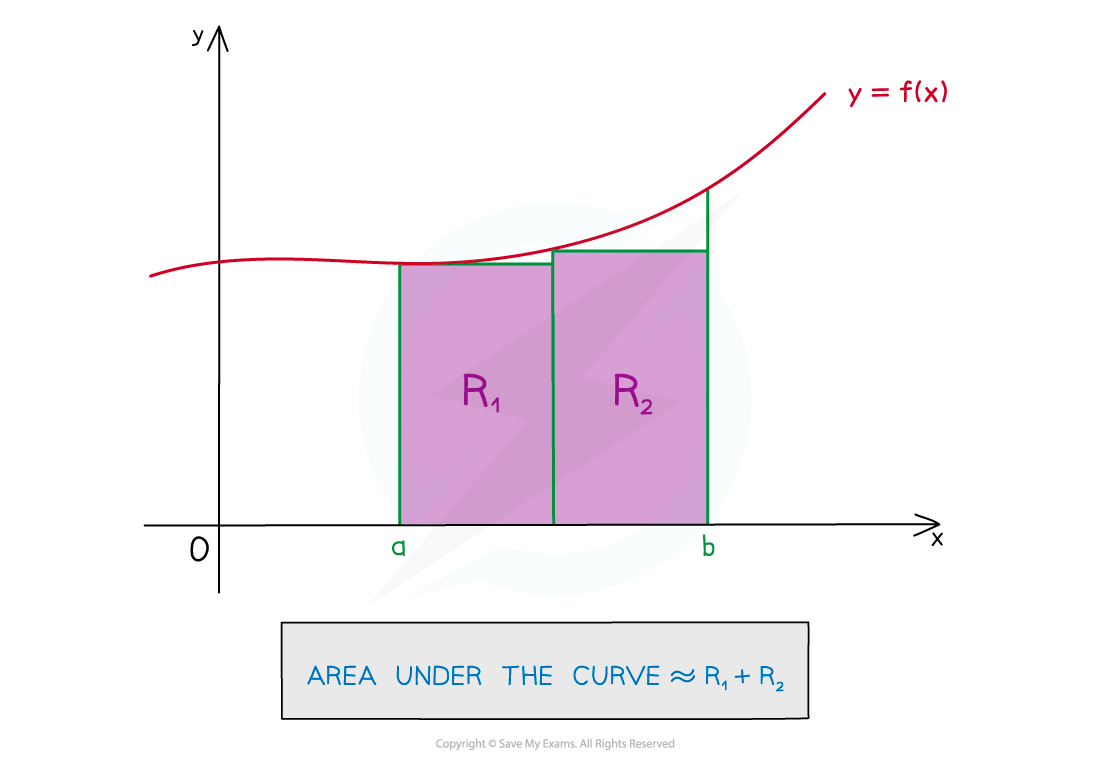• If the number of rectangles increases and their width decreases, the estimate is more accurate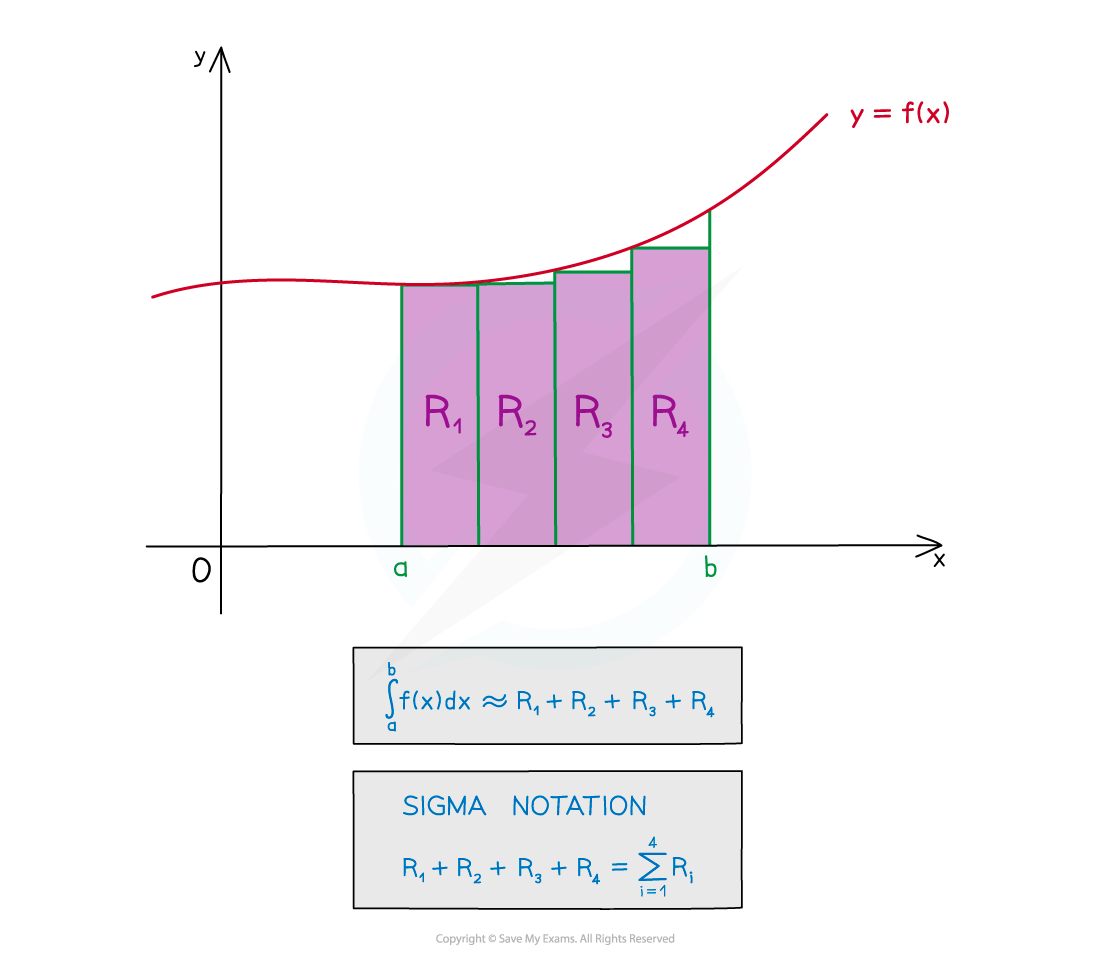• The sum of the rectangle areas will have a limit, however small they get
• The sum will become closer and closer to the area under the curve
• This is called the limit of the sum

#### What is integration as the limit of a sum?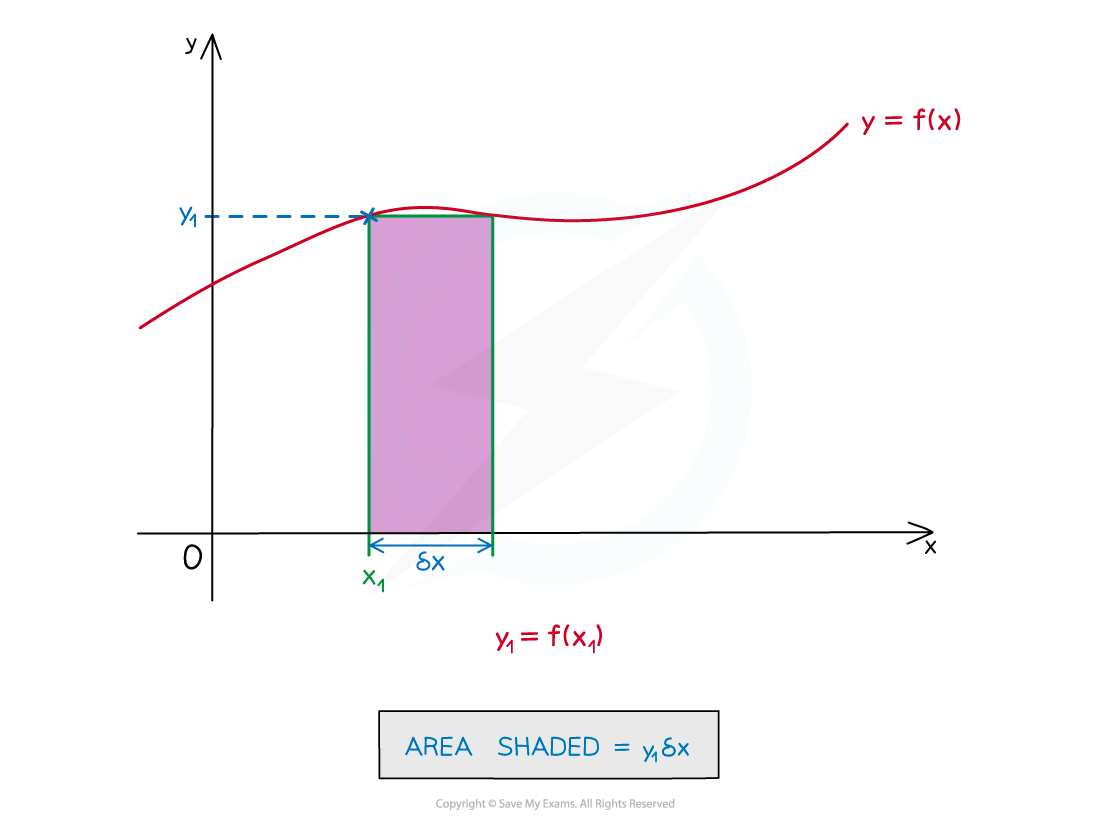• The width of a rectangle can be considered as a small increase along the x-axis
• This is denoted by δx
• The height (length) will be the y-coordinate at x1 – ie f(x1) (rather than f(x1+δx))
• If we use four of these small rectangles between a and b we get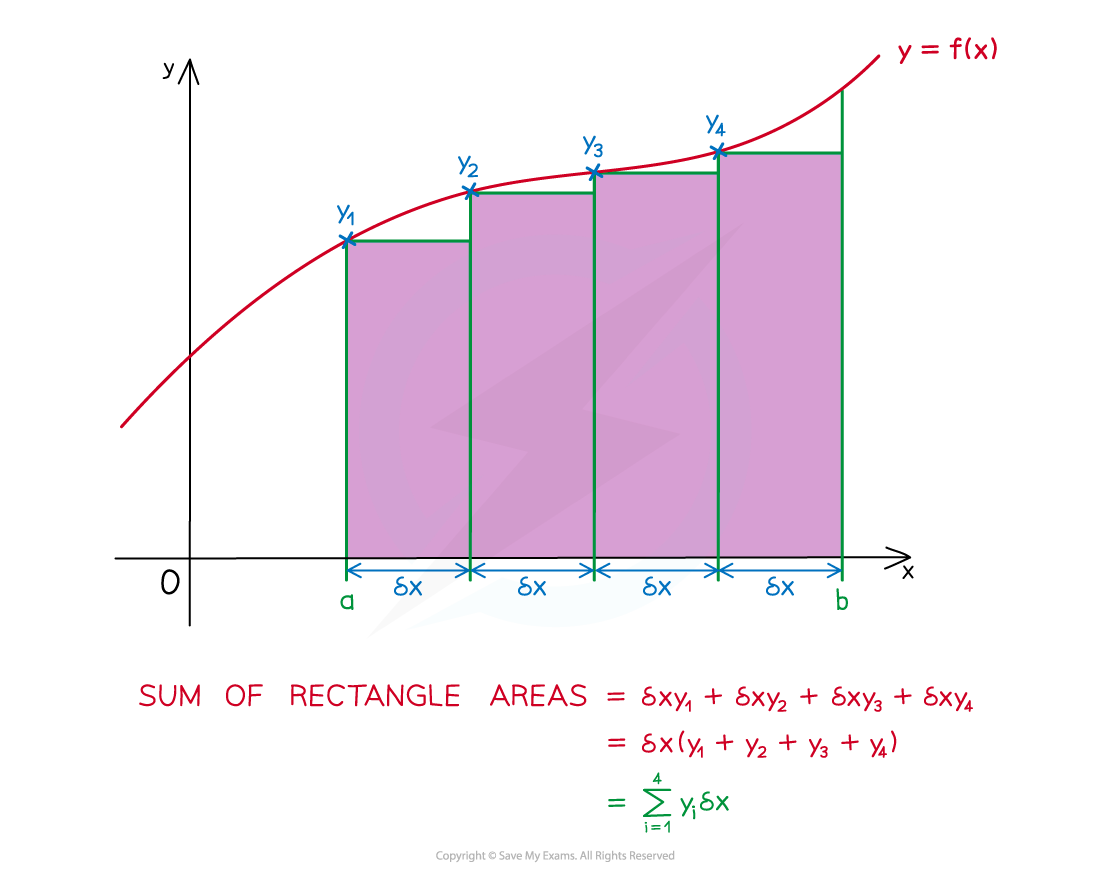• As more rectangles are used …
• … δx gets smaller and smaller, ie δx → 0
• … n, the number of rectangles, gets bigger and bigger, ie n → ∞
• … the sum of the area of the rectangles becomes closer to the area under the curve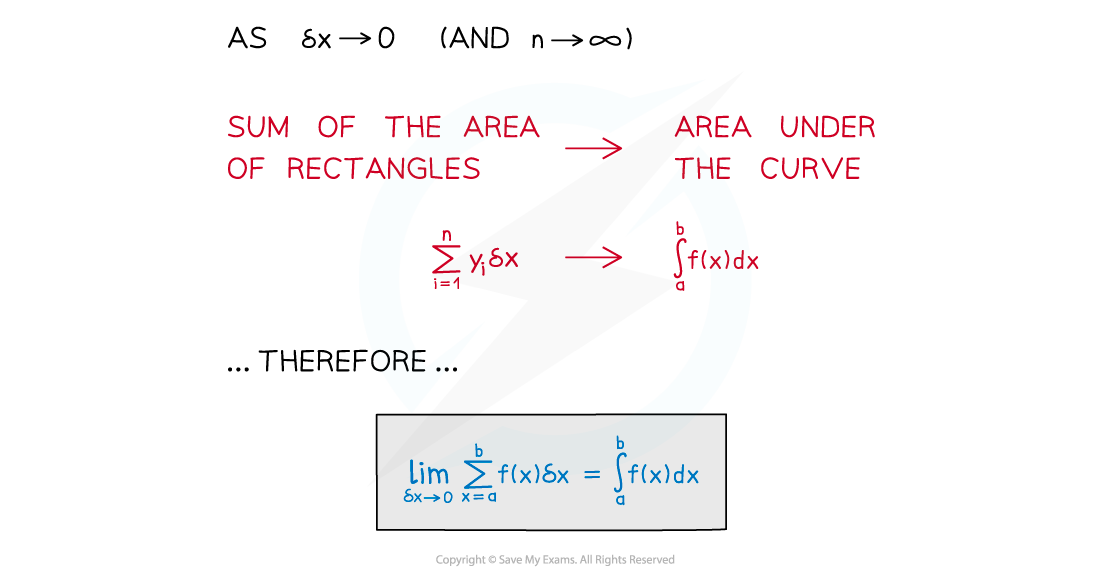• This is the meaning of integration as the limit of a sum

#### How do questions use integration as the limit of a sum?

• STEP 1        Recognise the notation
• STEP 2        Convert to a definite integral
• STEP 3        Find the value of the integral#### Worked Example